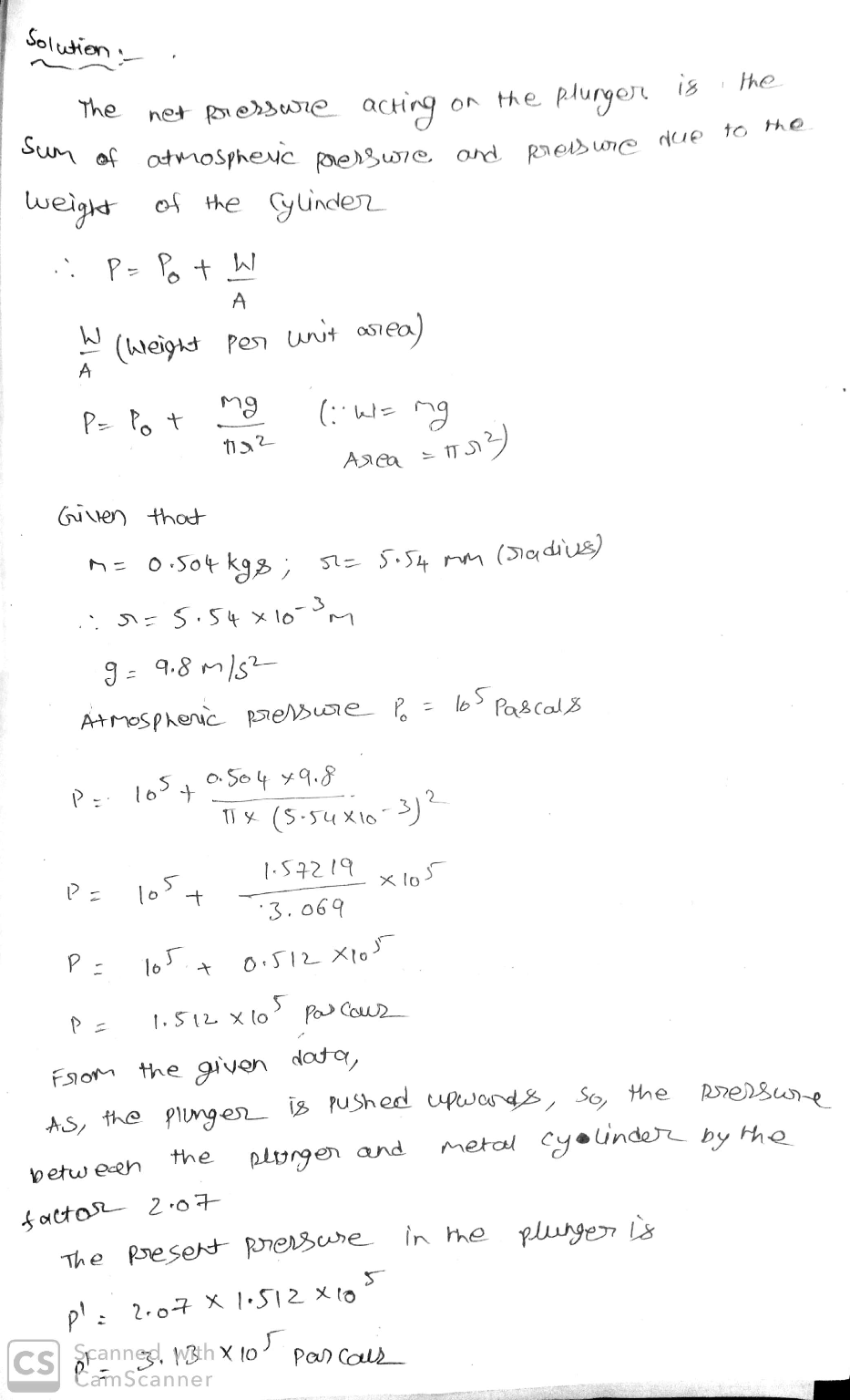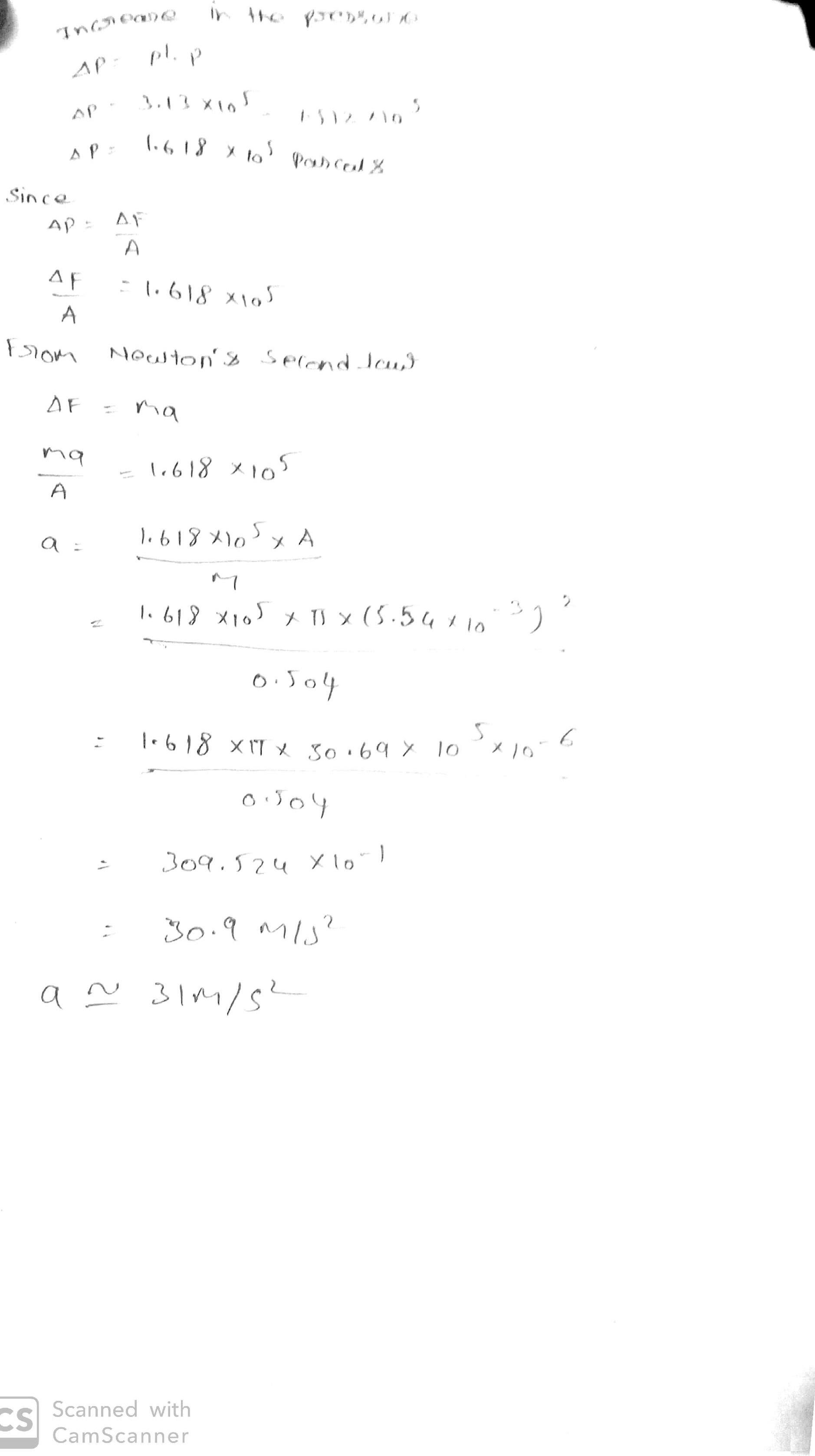In: Physics

# A 0.504 kg metal cylinder is placed inside the top of a plastic tube, the lower...

A 0.504 kg metal cylinder is placed inside the top of a plastic tube, the lower end of which is sealed off by an adjustable plunger. The cylinder comes to rest some distance above the plunger. The plastic tube has an inner radius of 5.54 mm and is frictionless. Neither the plunger nor the metal cylinder allow any air to flow around them. If the plunger is suddenly pushed upwards, increasing the pressure between the plunger and the metal cylinder by a factor of 2.07 , what is the initial acceleration a of the metal cylinder? Assume the pressure outside of the tube is 1.00 atm and that the top of the tube is open to the air.

## Solutions

##### Expert Solution## Related Solutions

##### Part A: A certain amount of chlorine gas was placed inside a cylinder with a movable...
Part A: A certain amount of chlorine gas was placed inside a cylinder with a movable piston at one end. The initial volume was 3.00 L and the initial pressure of chlorine was 1.80 atm . The piston was pushed down to change the volume to 1.00 L. Calculate the final pressure of the gas if the temperature and number of moles of chlorine remain constant.. Express your answer numerically in atmospheres. Part B: In an air-conditioned room at 19.0...
##### A metal cylinder with a mass of 3.50 kg is attached to a spring and is...
A metal cylinder with a mass of 3.50 kg is attached to a spring and is able to oscillate horizontally with negligible friction. The cylinder is pulled to a distance of 0.200 m from its equilibrium position, held in place with a force of 18.0 N,and then released from rest. It then oscillates in simple harmonic motion. (The cylinder oscillates along the x-axis, where x = 0 is the equilibrium position.) (a) What is the spring constant (in N/m)? N/m...
##### Two smooth spheres of radius are placed inside a cylinder of radius R. The mass of...
Two smooth spheres of radius are placed inside a cylinder of radius R. The mass of each sphere is M Assume that R < 2r < 2R All answers should be in terms of . R.M and What is the force of the bottom sphere on the top sphere? What is the force of the cylinder wall on the top sphere ?
##### Explain the procedure to generate a cylinder placed on top of a rectangular block using any...
Explain the procedure to generate a cylinder placed on top of a rectangular block using any CAD software.
##### A tube with a diameter of 6.45 mm was placed in a liquid with a density...
A tube with a diameter of 6.45 mm was placed in a liquid with a density of 998 kg / m3 and it was observed that the liquid increased by 1.44 mm in the tube. Find the surface tension of the fluid [N / m] by taking the contact angle 15 ° between the liquid and the tube surface.
##### The plastic portion of the lower mantle that supports the tectonic plates is called the ___________
The plastic portion of the lower mantle that supports the tectonic plates is called the ___________
##### A tube is placed vertically in a beaker of salt water with a specific gravity of...
A tube is placed vertically in a beaker of salt water with a specific gravity of 1.15. The interfacial tension between the air and the water is 32 mN/m and the contact angle is 30 degrees, calculate: a) Capillary pressure in psi if the tube diameter is 0.0001 cm. b) Capillary rise of water in tube. c) If the cap rock thickness is 2ft, do you think this will stop water migration? d) Radius in inches of the largest pore,...
##### A speaker is placed at the opening of a long horizontal tube. The speaker oscillates at...
A speaker is placed at the opening of a long horizontal tube. The speaker oscillates at a frequency of f creating a wave that moves down the tube. The wave moves through the tube at a speed of v=330 m/s.  At time t= 0s an air molecule at x=1m is at the maximum displacement of 5 nm. At the same time another molecule at x=3.7m has a displacement of 3.5nm. What is the wave function of the sound?
##### A tube has a diameter of 0.87 mm and is placed vertically in a liquid of...
A tube has a diameter of 0.87 mm and is placed vertically in a liquid of density 879 km/m^3, which rises 3.7 cm in the tube. The contact angle is 17 degrees between the liquid and tub. What is the surface tension of the liquid?
##### Two identical metal spheres A and B are connected by a plastic rod.
Two identical metal spheres A and B are connected by a plastic rod. Both are initially neutral. 4.0×1012 electrons are added to sphere A, then the connecting rod is removed. a)Afterward, what is the charge of A? b)Afterward, what is the charge of B?